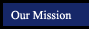Algebra 1 - Concepts, Skills and Problem Solving
Quadratic and Exponential Functions
Chapter 9
 Section Title Notebook files More examples (in pop-up window) 9.1 Graphing Quadratic Functions new per4 per6 notebook express 9.2 Solving Quadratic Equations by Graphing new per4 per6 notebook express 9.3 Solving Quadratic Equations by Completing the Square new per4 per6 notebook express 9.4 Solving Quadratic Equations by Using the Quadratic Formula new per4 per6 notebook express 9.5 Exponential Functions new per4 per6 notebook express 9.6 Growth and Decay new per4 per6 notebook express

Online Scientific Calculator from Calculator.com

Math Glossary
(more sophisticated than it looks!)